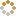﻿ N-B-U-T Online Judge ::  Nuclear Reactor
• ###  Nuclear Reactor

• 时间限制: 1000 ms　内存限制: 65535 K
• 问题描述
• A nuclear reactor have two types of events:
High-energy particle touch nuclear, released three high-energy particles and a low-energy particles;
Low-energy particle touch nuclear,released two high-energy particles and a low-energy particles;
Know that the first time(0 microsecond),there is only one high-energy particle in the nuclear reactor.Every touch cause a microsecond. So, how many particles in the nuclear reactor after n microseconds?

Every particle is still alive after touching.

• 输入
• The input will contain number 0 <= t<= 25 per line representing the number of response time and end of n=-1.
• 输出
• Output n microseconds moment the number of high-energy particles and the number of low-energy particles respectively, and separate them by “, ”. Each output of a line
• 样例输入
• ```5
2
-1
```
• 样例输出
• ```1872, 684
18, 6```
• 提示
• `无`
• 来源
• `Silvar`
• 操作
﻿